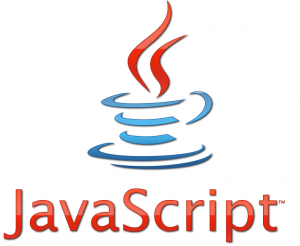# oDesk JavaScript Test1) What is the final value of the variable bar in the following code?
var foo = 9;
bar = 5;
(function() {
var foo = 2;
bar= 1;
}())
bar = bar + foo;

```(Answer) bar = 10
```

2) What is the meaning of obfuscation in JavaScript?

```(Answer) Making code unreadable using advanced algorithms.
```

3) Which of the following is not a valid HTML event?

```(Answer) onblink
```

4) What value would JavaScript assign to an uninitialized variable?

```(Answer) undefined
```

5) What will be output of the following code?
function testGenerator() {
yield “first”;
document.write(“step1”);

yield “second”;
document.write(“step2”);

yield “third”;
document.write(“step3”);
}
var g = testGenerator();
document.write(g.next());
document.write(g.next());

```(Answer) firststep1second
```

6) What will be the final value of the variable “apt”?
var apt=2;
apt=apt<<2;

```(Answer) 8
```

7) What would be the use of the following code?
function validate(field) {
var valid=”ABCDEFGHIJKLMNOPQRSTUVWXYZabcdefghijklmnopqrstuvwxyz”;
var ok=”yes”;
var temp;

for(var i=0;i (Answer) It will force a user to enter only English alphabet character values. 8) What would be the value of ‘ind’ after execution of the following code? var msg=”Welcome to ExpertRating” var ind= msg.substr(3, 3)

```(Answer) com
```

9) When setting cookies with JavaScript, what will happen to the cookies.txt data if the file exceeds the maximum size?

```(Answer) The file is truncated to the maximum length.
```

10) Which event can be used to validate the value in a field as soon as the user moves out of the field by pressing the tab key?

```(Answer) onblur
```

11) Which object can be used to ascertain the protocol of the current URL?

```(Answer) location
```

12) Which of the following are correct values of variableC, and why?
` <script> variableA = [6,8]; variableB =[7,9]; variableC = variableA + variableB; </script> `

```(Answer) 6, 87 and 9. The + operator is not defined for arrays, and it concatenates strings, so it converts the arrays to strings.
```

13) Which of the following choices will turn a string into a JavaScript function call (case with objects) of the following code snippet?
` <script> window.foo = { bar: { baz: function() { alert('Hello!'); } } }; </script>; `

```(Answer) window['foo']['bar']['baz']();
```

14) Which of the following are JavaScript unit testing tools?

```(Answer) Buster.js, YUI Yeti, Jasmine
```

15) Which of the following are not global methods and properties in E4X?

```(Answer) setName() and setNamespace()
```

16) Which of the following Array methods in JavaScript runs a function on every item in the Array and collects the result from previous calls, but in reverse?

```(Answer) reduceRight()
```

17) Which of the following best describes a “for” loop?

```(Answer) "for" loop consists of three optional expressions, enclosed in parentheses and separated by semicolons, followed by a statement executed in the loop.
```

18) Which of the following built-in functions is used to access form elements using their IDs?

```(Answer) getElementById(id)
```

19) Which of the following can be used for disabling the right click event in Internet Explorer?

```(Answer) event.button == 2
```

20) Which of the following can be used to escape the ‘ character?

```(Answer) \
```

21) Which of the following can be used to invoke an iframe from a parent page?

```(Answer) windows.frames
```

22) Which of the following choices will change the source of the image to “image2.gif” when a user clicks on the image?

```(Answer) img id="imageID" src="image1.gif" width="50" height="60" onmousedown="changeimg(image1.gif)" onmouseup="changeimg(image2.gif)"
```

23) Which of the following choices will detect if “variableName” declares a function?
` <script> var variableName= function(){}; </script> `

```(Answer) typeof variableName;
```

24) What is the purpose of while(1) in the following JSON response?
` while(1);[['u',[['smsSentFlag','false'],['hideInvitations','false'],['remindOnRespondedEventsOnly','true'],['hideInvitations_remindOnRespondedEventsOnly','false_true'],['Calendar ID stripped for privacy','false'],['smsVerifiedFlag','true']]]] `

```(Answer) It makes it difficult for a third-party to insert the JSON response into an HTML document with a tag.
```
1.8 years ago